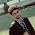# TCS NQT C-MCQ Set - 5

Ques 1. Eesha wrote a recursive function that takes the first node in a linked list as an argument, reverses the list, returning the first Node in the result. The pseudo code for this function is given below. However, she did not get the correct result. In which line number did she make a mistake?

public Node  reverse(Node first)
{
if (first == null)   return null;
if (first.next == null)   return first;
Node second = first.next;
Node rest  = reverse (second);
second.next = first;
first.next = null;
return rest.next;
}

Ques. 2 What will happen if in a C program you assign a value to an array element whose subscript exceeds the size of array?

A. The element will be set to 0.
B. The compiler would report an error.
C. The program may crash if some important data gets overwritten. ✔️
D. The array size would appropriately grow

Explanation: If the index of the array size is exceeded, the program will crash. Hence “option c” is the correct answer. But the modern compilers will take care of this kind of errors.

Ques 3. What does the following declaration mean?

int (*ptr);

A. ptr is array of pointers to 10 integers
B. ptr is a pointer to an array of 10 integers  ✔️
C. ptr is an array of 10 integers
D. ptr is an pointer to array

Ques 4.  In C, if you pass an array as an argument to a function, what actually gets passed?

A. Value of elements in array
B. First element of the array
C. Base address of the array ✔️
D. Address of the last element of array

Ques 5.  What will be the output of the program?

#include<stdio.h>
int main()
{
int a = {5, 1, 15, 20, 25};
int i, j, m;
i = ++a;
j = a++;
m = a[i++];
printf("%d, %d, %d", i, j, m);
return 0;
}

A. 2, 1, 15
B. 1, 2, 5
C. 3, 2, 15  ✔️
D. 2, 3, 20

Explanation:
Step 1: int a = {5, 1, 15, 20, 25}; The variable arr is declared as an integer array with a size of 5 and it is initiapzed to
a = 5, a = 1, a = 15, a = 20, a = 25 .
Step 2: int i, j, m; The variable i,j,m are declared as an integer type.
Step 3: i = ++a; becomes i = ++1; Hence i = 2 and a = 2
Step 4: j = a++; becomes j = 2++; Hence j = 2 and a = 3.
Step 5: m = a[i++]; becomes m = a; Hence m = 15 and i is incremented by 1(i++ means 2++ so i=3)
Step 6: printf(“%d, %d, %d”, i, j, m); It prints the value of the variables i, j, m
Hence the output of the program is 3, 2, 15

Ques 6. Is there any difference int the following declarations?

int fun(int arr[]);

int fun(int arr);

A. Yes
B. No  ✔️

Explanation:  No, both the statements are the same. It is the prototype for the function fun() that accepts one integer array as a parameter and returns an integer value.

Ques 7. Are the expressions arr and &arr same for an array of 10 integers?

A.Yes
B. No  ✔️

Explanation: Both mean two different things. arr gives the address of the first int, whereas the &arr gives the address of array of ints

Ques 8. Which of the fplowing statements should be used to obtain a remainder after dividing 3.14 by 2.1?

A. rem = 3.14 % 2.1;
B. rem = modf(3.14, 2.1);
C. rem = fmod(3.14, 2.1); ✔️
D. Remainder cannot be obtain in floating point division.

Explanation:
fmod(x,y) – Calculates x modulo y, the remainder of x/y.
This function is the same as the modulus operator. But fmod() performs floating point divisions.

See more TCS NQT (National Qualifier Test) C-MCQ  Questions

TCS C-MCQ Programming Set-2

TCS C-MCQ Programming Set-3

TCS C-MCQ Programming Set-4

TCS C-MCQ Programming Set-51.1.